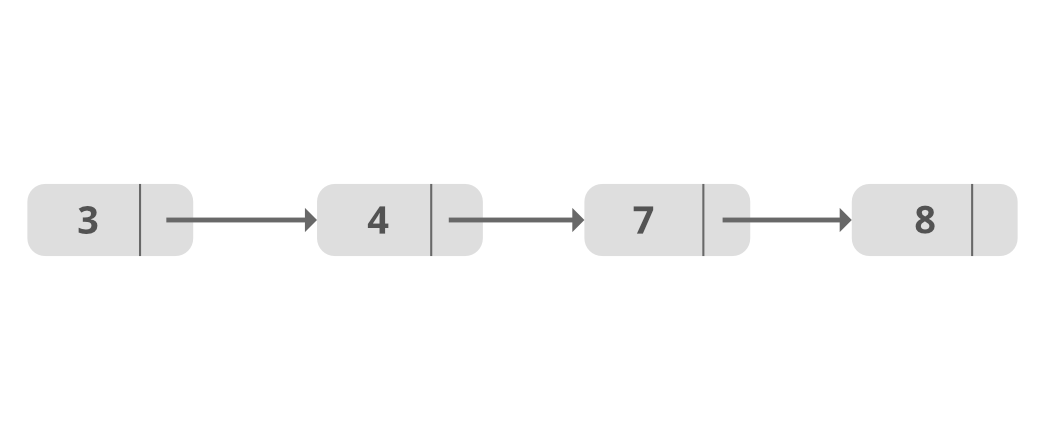How to Sort a LinkedList in Java?

• Last Updated : 20 Sep, 2021

A Linked List is a linear data structure, in which the elements are not stored at contiguous memory locations.

Sorting the nodes of a Singly Linked list in ascending order:Original ListSorted List

We can sort the LinkedList by many sorting techniques:

1. Bubble sort
2. Insertion sort
3. Quick sort
4. Merge sort

Method 1: Sort using Bubble Sort

• To accomplish this task, we maintain two pointers: current and index.
• Initially, current point to head node and index will point to node next to current.
• Traverse through the list till current points to null, by comparing current’s data with index’s data.
• And for each current’s value, index is the next to current node which traverse from current’s next node till null.
• And then the value of current node is compared with every value from its next node till last and if the value is smaller than the current value, then the values are swapped and in this way the least value comes as current index.

Java

Output
Original list:
8 3 7 4
Sorted list:
3 4 7 8

Time complexity: O(n ^ 2)

Space complexity: O(1)

Method 2: Sort using Insertion Sort

• In the Insertion sort technique, we assume that all the elements before the current element in the list is already sorted, and we begin with the current element.
• The current element is compared with all the elements before it and swapped if not in order. This process is repeated for all the subsequent elements.
• In general, the Insertion sort technique compares each element with all of its previous elements and sorts the element to place it in its proper position.

As already mentioned, the Insertion sort technique is more feasible for a smaller set of data, and thus arrays with a few elements can be sorted using efficiently Insertion sort.

Insertion sort is especially useful in sorting linked list data structures. As you know, Linked lists have pointers pointing to its next element (singly linked list) and previous element (double linked list). This makes it easier to keep track of the previous and next elements.

Java

Output
8 3 7 4
3 4 7 8

Time complexity: O(n ^ 2)

Space complexity: O(1)

Method 3: Sort using Quick Sort

Quick sort follows divide and conquer approach. It picks an element as pivot and partitions the given array around the picked pivot.

The key process in quickSort is partition(). Target of partitions is, given an array and an element x of array as pivot, put x at its correct position in sorted array and put all smaller elements (smaller than x) before x, and put all greater elements (greater than x) after x. All this should be done in linear time.

Quick sort is preferred over merge sort as Quick sort is an in-place algorithm (meaning, no additional memory space required).

Java

Output
Original List:
8 3 7 4
Sorted List:
3 4 7 8

Time complexity: O(n ^ 2)

Space complexity: O(1)

Method 3: Sort using Merge Sort

Merge sort is often preferred for sorting a linked list. The slow random-access performance of a linked list makes some other algorithms (such as quicksort) perform poorly, and others (such as heapsort) completely impossible.

Merge Sort is a Divide and Conquer algorithm. It divides the input array into two halves, calls itself for the two halves, and then merges the two sorted halves. The merge() function is used for merging two halves. The merge(arr, l, m, r) is a key process that assumes that arr[l..m] and arr[m+1..r] are sorted and merges the two sorted sub-arrays into one.

• Note that we need a reference to head in MergeSort() as the below implementation changes next links to sort the linked lists (not data at the nodes), so head node has to be changed if the data at original head is not the smallest value in linked list.

Java

Output
Original List:
8 3 7 4
Sorted List:
3 4 7 8

Time complexity: O(n log n)

Space complexity: O(1)

My Personal Notes arrow_drop_up